# Expectation Maximization¶

The Expectation Maximization(EM) algorithm estimates the parameters of the multivariate probability density function in the form of a Gaussian mixture distribution with a specified number of mixtures.

Consider the set of the N feature vectors {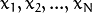} from a d-dimensional Euclidean space drawn from a Gaussian mixture: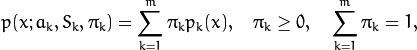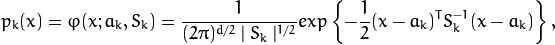where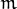is the number of mixtures,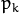is the normal distribution density with the mean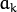and covariance matrix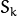,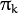is the weight of the k-th mixture. Given the number of mixtures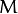and the samples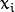,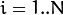the algorithm finds the maximum-likelihood estimates (MLE) of all the mixture parameters, that is,,and: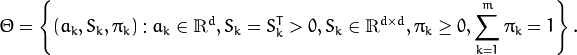The EM algorithm is an iterative procedure. Each iteration includes two steps. At the first step (Expectation step or E-step), you find a probability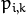(denoted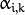in the formula below) of sample i to belong to mixture k using the currently available mixture parameter estimates: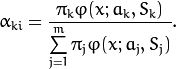At the second step (Maximization step or M-step), the mixture parameter estimates are refined using the computed probabilities: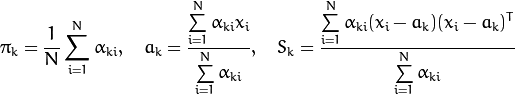Alternatively, the algorithm may start with the M-step when the initial values forcan be provided. Another alternative whenare unknown is to use a simpler clustering algorithm to pre-cluster the input samples and thus obtain initial. Often (including machine learning) the kmeans() algorithm is used for that purpose.

One of the main problems of the EM algorithm is a large number of parameters to estimate. The majority of the parameters reside in covariance matrices, which are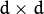elements each where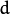is the feature space dimensionality. However, in many practical problems, the covariance matrices are close to diagonal or even to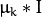, whereis an identity matrix and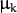is a mixture-dependent “scale” parameter. So, a robust computation scheme could start with harder constraints on the covariance matrices and then use the estimated parameters as an input for a less constrained optimization problem (often a diagonal covariance matrix is already a good enough approximation).

References:

• Bilmes98 J. A. Bilmes. A Gentle Tutorial of the EM Algorithm and its Application to Parameter Estimation for Gaussian Mixture and Hidden Markov Models. Technical Report TR-97-021, International Computer Science Institute and Computer Science Division, University of California at Berkeley, April 1998.

## EM¶

class EM : public Algorithm

The class implements the EM algorithm as described in the beginning of this section. It is inherited from Algorithm.

## EM::EM¶

The constructor of the class

C++: EM::EM(int nclusters=EM::DEFAULT_NCLUSTERS, int covMatType=EM::COV_MAT_DIAGONAL, const TermCriteria& termCrit=TermCriteria(TermCriteria::COUNT+TermCriteria::EPS, EM::DEFAULT_MAX_ITERS, FLT_EPSILON) )
Python: cv2.EM([nclusters[, covMatType[, termCrit]]]) → <EM object>
Parameters: nclusters – The number of mixture components in the Gaussian mixture model. Default value of the parameter is EM::DEFAULT_NCLUSTERS=5. Some of EM implementation could determine the optimal number of mixtures within a specified value range, but that is not the case in ML yet. covMatType – Constraint on covariance matrices which defines type of matrices. Possible values are: EM::COV_MAT_SPHERICAL A scaled identity matrix. There is the only parameterto be estimated for each matrix. The option may be used in special cases, when the constraint is relevant, or as a first step in the optimization (for example in case when the data is preprocessed with PCA). The results of such preliminary estimation may be passed again to the optimization procedure, this time with covMatType=EM::COV_MAT_DIAGONAL. EM::COV_MAT_DIAGONAL A diagonal matrix with positive diagonal elements. The number of free parameters is d for each matrix. This is most commonly used option yielding good estimation results. EM::COV_MAT_GENERIC A symmetric positively defined matrix. The number of free parameters in each matrix is about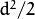. It is not recommended to use this option, unless there is pretty accurate initial estimation of the parameters and/or a huge number of training samples. termCrit – The termination criteria of the EM algorithm. The EM algorithm can be terminated by the number of iterations termCrit.maxCount (number of M-steps) or when relative change of likelihood logarithm is less than termCrit.epsilon. Default maximum number of iterations is EM::DEFAULT_MAX_ITERS=100.

## EM::train¶

Estimates the Gaussian mixture parameters from a samples set.

C++: bool EM::train(InputArray samples, OutputArray logLikelihoods=noArray(), OutputArray labels=noArray(), OutputArray probs=noArray())
C++: bool EM::trainE(InputArray samples, InputArray means0, InputArray covs0=noArray(), InputArray weights0=noArray(), OutputArray logLikelihoods=noArray(), OutputArray labels=noArray(), OutputArray probs=noArray())
C++: bool EM::trainM(InputArray samples, InputArray probs0, OutputArray logLikelihoods=noArray(), OutputArray labels=noArray(), OutputArray probs=noArray())
Python: cv2.EM.train(samples[, logLikelihoods[, labels[, probs]]]) → retval, logLikelihoods, labels, probs
Python: cv2.EM.trainE(samples, means0[, covs0[, weights0[, logLikelihoods[, labels[, probs]]]]]) → retval, logLikelihoods, labels, probs
Python: cv2.EM.trainM(samples, probs0[, logLikelihoods[, labels[, probs]]]) → retval, logLikelihoods, labels, probs
Parameters: samples – Samples from which the Gaussian mixture model will be estimated. It should be a one-channel matrix, each row of which is a sample. If the matrix does not have CV_64F type it will be converted to the inner matrix of such type for the further computing. means0 – Initial meansof mixture components. It is a one-channel matrix of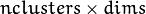size. If the matrix does not have CV_64F type it will be converted to the inner matrix of such type for the further computing. covs0 – The vector of initial covariance matricesof mixture components. Each of covariance matrices is a one-channel matrix of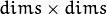size. If the matrices do not have CV_64F type they will be converted to the inner matrices of such type for the further computing. weights0 – Initial weightsof mixture components. It should be a one-channel floating-point matrix with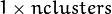or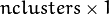size. probs0 – Initial probabilitiesof sampleto belong to mixture component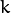. It is a one-channel floating-point matrix of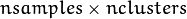size. logLikelihoods – The optional output matrix that contains a likelihood logarithm value for each sample. It has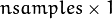size and CV_64FC1 type. labels – The optional output “class label” for each sample: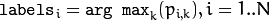(indices of the most probable mixture component for each sample). It hassize and CV_32SC1 type. probs – The optional output matrix that contains posterior probabilities of each Gaussian mixture component given the each sample. It hassize and CV_64FC1 type.

Three versions of training method differ in the initialization of Gaussian mixture model parameters and start step:

• train - Starts with Expectation step. Initial values of the model parameters will be estimated by the k-means algorithm.
• trainE - Starts with Expectation step. You need to provide initial meansof mixture components. Optionally you can pass initial weightsand covariance matricesof mixture components.
• trainM - Starts with Maximization step. You need to provide initial probabilitiesto use this option.

The methods return true if the Gaussian mixture model was trained successfully, otherwise it returns false.

Unlike many of the ML models, EM is an unsupervised learning algorithm and it does not take responses (class labels or function values) as input. Instead, it computes the Maximum Likelihood Estimate of the Gaussian mixture parameters from an input sample set, stores all the parameters inside the structure:in probs,in means ,in covs[k],in weights , and optionally computes the output “class label” for each sample:(indices of the most probable mixture component for each sample).

The trained model can be used further for prediction, just like any other classifier. The trained model is similar to the CvNormalBayesClassifier.

## EM::predict¶

Returns a likelihood logarithm value and an index of the most probable mixture component for the given sample.

C++: Vec2d EM::predict(InputArray sample, OutputArray probs=noArray()) const
Python: cv2.EM.predict(sample[, probs]) → retval, probs
Parameters: sample – A sample for classification. It should be a one-channel matrix of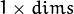or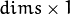size. probs – Optional output matrix that contains posterior probabilities of each component given the sample. It hassize and CV_64FC1 type.

The method returns a two-element double vector. Zero element is a likelihood logarithm value for the sample. First element is an index of the most probable mixture component for the given sample.

## CvEM::isTrained¶

Returns true if the Gaussian mixture model was trained.

C++: bool EM::isTrained() const
Python: cv2.EM.isTrained() → retval

## EM::get, EM::set¶

See Algorithm::get() and Algorithm::set(). The following parameters are available:

• "nclusters"
• "covMatType"
• "maxIters"
• "epsilon"